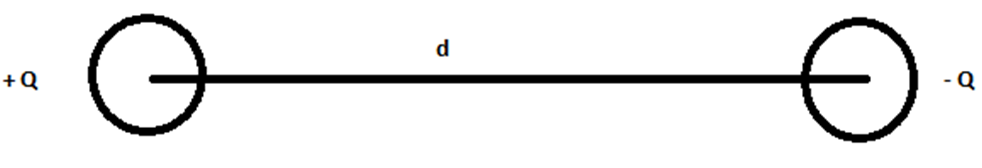# Electric Dipole

## What is Electric Dipole?

An electric dipole is defined as a couple of opposite charges q and –q separated by a distance d. By default, the direction of electric dipoles in space is always from negative charge -q to positive charge q. The midpoint q and –q is called the centre of the dipole. The simplest example of an electric dipole is a pair of electric charges of two opposite signs and equal magnitude separated by distance.

## Visualizing Electric Dipole

Suppose there are two charges of equal magnitude ‘q’, separated by a distance, d. Let the first charge be negative and the second charge is positive. This combination can be called an electric dipole. Therefore, we can say that an electric dipole is created by the combination of equal and opposite charges by a separation of a certain distance.An electric dipole is denoted by the symbol

$$\begin{array}{l}\vec{p}\end{array}$$
. We know that electric dipole is the product of the magnitude of the charges multiplied by the distance between them., and this can be mathematically represented as follows:

### The magnitude of an electric dipole is given as:

$$\begin{array}{l} \overrightarrow{p} \end{array}$$
=
$$\begin{array}{l} Q \overrightarrow {d} \end{array}$$

### Direction of Electric Dipole Moment

The electric dipole moment is a vector quantity; it has a defined direction which is from the negative charge to the positive charge. Though, it is important to remember that this convention of direction is only followed in Physics. In Chemistry, the convention is taken to be opposite i.e. from positive to negative. The line along the direction of an electric dipole is called the axis of the dipole.

You may also want to check out these topics given below!

### Electric potential due to a Dipole (V)

Suppose there are two charges –q, placed at A, and +q placed a B, separated by a distance d, forming a dipole. Suppose the midpoint of AB is O.

The Electric potential due to a dipole at any point P, such that OP = r will be:

 V = $$\begin{array}{l}\frac{1}{4\pi \epsilon } \frac{p cos \theta }{r^{2}}\end{array}$$

Case 1: If θ = 90°

 Electric potential = V = 0

Case 2: If θ = 0°

 Electric potential = V = $$\begin{array}{l}\frac{1}{4\pi \epsilon } \frac{p }{r^{2}}\end{array}$$

## Physical Significance of Dipole

Electric dipole is not only prominent in electrostatics but also in chemistry. In most molecules the centre of positive and negative charges coincide at the same point because of which the distance between two charges is zero. Carbon dioxide and methane fall under the category of zero dipole moment. This type of molecules are known as non-polar molecules. The molecules that have permanent dipole moment as the centre of positive and negative charge don’t coincide are called polar molecules.

If you would like to read more about polar and non-polar molecules, click on the link below:

## Frequently Asked Questions – FAQs

### What is the net force acting on a dipole placed in a uniform electric field?

The forces on the two charges constituting the dipole are equal and opposite. Hence, the net force is zero.

### What is the SI unit of the dipole moment?

The SI unit of dipole moment is Coulomb.meter

### When is the torque on a dipole maximum?

When the dipole is held perpendicular to the field, the torque is maximum.

### When is the torque on a dipole minimum?

When the dipole is parallel to the field, the torque on a dipole is minimum.

### Give an example of the electric dipole.

A pair of electric charge of two opposite signs and equal magnitude separated by a distance.

Stay tuned with BYJU’S to learn more Physics concepts with the help of interactive video lessons.

Test Your Knowledge On Electric Dipole!

#### 1 Comment

1. Super 👌 explanation